# 1448 Christmas Factor Tree

Here’s a puzzle that looks a little like a Christmas tree. Some of the clues might give you a little bit of trouble. For example, the common factor of 60 and 30 might be 5, 6, or 10. Likewise, the common factor of 8 and 4 might be 1, 2, or 4.

Which factor should you use? Look at all the other clues and use logic. Logic can help you write each of the numbers 1 to 12 in both the first column and the top row so that the given clues and those numbers behave like a multiplication table. Good luck!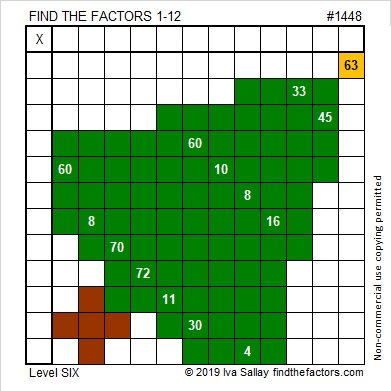I have to number every puzzle. It won’t help you solve the puzzle, but here are some facts about the number 1448:

The number made by its last two digits, 48, is divisible by 4, so 1448 is also divisible by 4. That fact can give us the first couple of branches of 1448’s factor tree: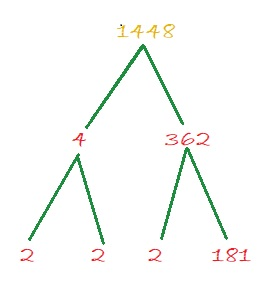• 1448 is a composite number.
• Prime factorization: 1448 = 2 × 2 × 2 × 181, which can be written 1448 = 2³ × 181
• 1448 has at least one exponent greater than 1 in its prime factorization so √1448 can be simplified. Taking the factor pair from the factor pair table below with the largest square number factor, we get √1448 = (√4)(√362) = 2√362
• The exponents in the prime factorization are 3 and 1. Adding one to each exponent and multiplying we get (3 + 1)(1 + 1) = 4 × 2 = 8. Therefore 1448 has exactly 8 factors.
• The factors of 1448 are outlined with their factor pair partners in the graphic below.1448 is also the hypotenuse of a Pythagorean triple:
152-1440-1448 which is 8 times (19-180-181)

# 1339 Written Eligible Factor Pairs Can Reveal the Needed Logic

The logic needed for this particular level 6 puzzle is VERY complicated so I wrote out the eligible factor pairs of all the clues for you.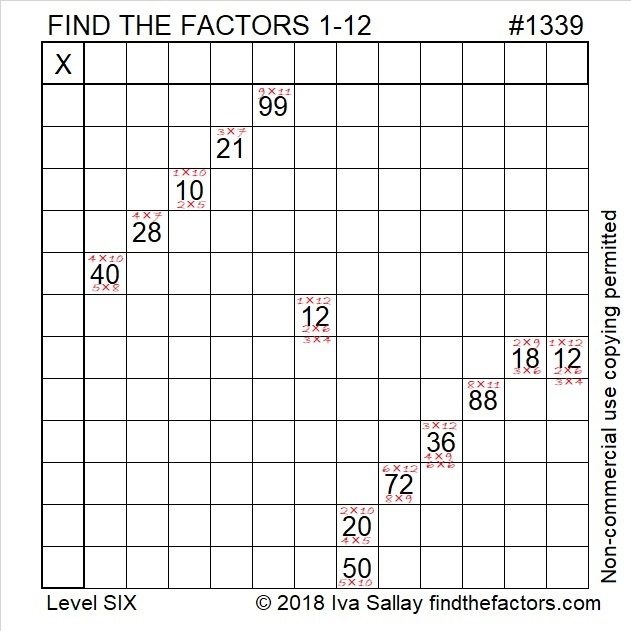Or you can start from scratch:Print the puzzles or type the solution in this excel file: 12 factors 1333-1341

Here are a few facts about the puzzle number, 1339:

• 1339 is a composite number.
• Prime factorization: 1339 = 13 × 103
• 1339 has no exponents greater than 1 in its prime factorization, so √1339 cannot be simplified.
• The exponents in the prime factorization are 1, and 1. Adding one to each exponent and multiplying we get (1 + 1)(1 + 1) = 2 × 2 = 4. Therefore 1339 has exactly 4 factors.
• The factors of 1339 are outlined with their factor pairs in the graphic below.1339 is the hypotenuse of a Pythagorean triple:
515-1236-1339 which is (5-12-13) times 103

# 1062 Complicated Logic

The logic used to solve this particular level 6 puzzle is complicated, but answering the following questions in the order given will help you to see and understand that logic:

1. Two of the numbers from 1 to 10 have only one clue each in this puzzle. What are those two numbers? The product of those two numbers is the missing clue that you will use later in the puzzle.
2. Which two clues MUST use both 1’s?
3. Which four clues must use all the 3’s and 6’s?
4. Can both 30’s be 3 × 10 or be 5 × 6?
5. Can both 40’s be 4 × 10 or be 5 × 8?
6. What MUST be the factors of 24 in this puzzle?
7. What clues must use both 4’s? What clues must use both 8s?
8. Is 1 or 2 the common factor for clues 8 and 2 that will make the puzzle work?
9. Is 5 or 10 the common factor for clues 30 and 40 near the bottom of the puzzle?

Once you know the answers to those questions and the two sets of common factors, you can very quickly complete the puzzle.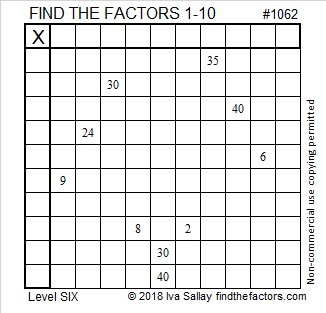Print the puzzles or type the solution in this excel file: 10-factors-1054-1062

Here’s some information about the number 1062:

• 1062 is a composite number.
• Prime factorization: 1062 = 2 × 3 × 3 × 59, which can be written 1062 = 2 × 3² × 59
• The exponents in the prime factorization are 1, 2, and 1. Adding one to each and multiplying we get (1 + 1)(2 + 1)(1 + 1) = 2 × 3 × 2 = 12. Therefore 1062 has exactly 12 factors.
• Factors of 1062: 1, 2, 3, 6, 9, 18, 59, 118, 177, 354, 531, 1062
• Factor pairs: 1062 = 1 × 1062, 2 × 531, 3 × 354, 6 × 177, 9 × 118, or 18 × 59,
• Taking the factor pair with the largest square number factor, we get √1062 = (√9)(√118) = 3√118 ≈ 32.58834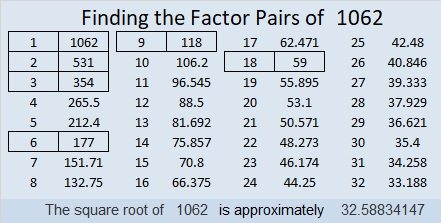1062 looks interesting when it is written in a couple of different bases:
It’s 2D2 in BASE 20 (D is 13 base 10) because 2(20²) + 13(20) + 2(1) = 1062
and 246 in BASE 22 because 2(22²) + 4(22) + 6(1) = 1062

# 597 and Level 5

597 is made from three consecutive odd numbers so it is divisible by 3.

Because it is divisible by 3, it is the sum of three consecutive numbers. It is also the sum of three consecutive odd numbers:

• 198 + 199 + 200 = 597
• 197 + 199 + 201 = 597

Why was 199 the middle number in both of those sums?

OEIS.org informs us that even though (597!!! + 1) generates an error message on our calculators, it is indeed a very large prime number. Whoever discovered that fact must have been extremely excited.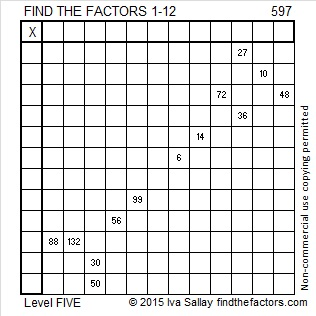Print the puzzles or type the solution on this excel file: 12 Factors 2015-08-24

—————————————————————————————————

• 597 is a composite number.
• Prime factorization: 597 = 3 x 199
• The exponents in the prime factorization are 1 and 1. Adding one to each and multiplying we get (1 + 1)(1 + 1) = 2 x 2 = 4. Therefore 597 has exactly 4 factors.
• Factors of 597: 1, 3, 199, 597
• Factor pairs: 597 = 1 x 597 or 3 x 199
• 597 has no square factors that allow its square root to be simplified. √597 ≈ 24.43358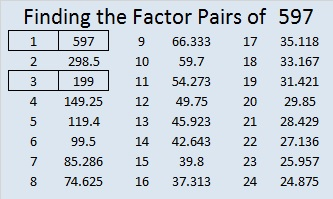—————————————————————————————————# 564 and Level 6

Consecutive primes 281 and 283 add up to 564.

564 is made from three consecutive numbers so it can be evenly divided by 3. If the middle number is divisible by 3, then a number made from three consecutive numbers will also be divisible by 9. Is 564 divisible by 9? Why or why not?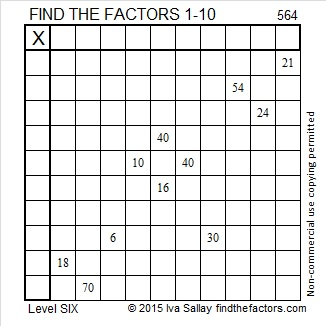Print the puzzles or type the solution on this excel file: 10 Factors 2015-07-20

—————————————————————————————————

• 564 is a composite number.
• Prime factorization: 564 = 2 x 2 x 3 x 47, which can be written 564 = (2^2) x 3 x 47
• The exponents in the prime factorization are 2, 1, and 1. Adding one to each and multiplying we get (2 + 1)(1 + 1)(1 + 1) = 3 x 2 x 2 = 12. Therefore 564 has exactly 12 factors.
• Factors of 564: 1, 2, 3, 4, 6, 12, 47, 94, 141, 188, 282, 564
• Factor pairs: 564 = 1 x 564, 2 x 282, 3 x 188, 4 x 141, 6 x 94, or 12 x 47
• Taking the factor pair with the largest square number factor, we get √564 = (√4)(√141) = 2√141 ≈ 23.74868—————————————————————————————————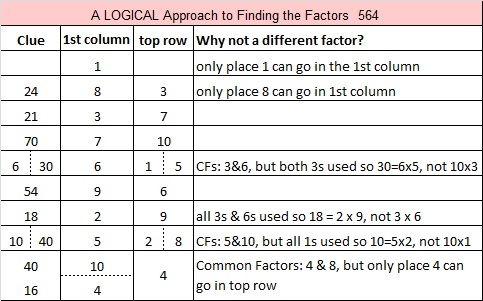# 563 and Level 5

563 is prime, and it is the sum of all the prime numbers from 5 to 67.Print the puzzles or type the solution on this excel file: 10 Factors 2015-07-20

—————————————————————————————————

• 563 is a prime number.
• Prime factorization: 563 is prime.
• The exponent of prime number 563 is 1. Adding 1 to that exponent we get (1 + 1) = 2. Therefore 563 has exactly 2 factors.
• Factors of 563: 1, 563
• Factor pairs: 563 = 1 x 563
• 563 has no square factors that allow its square root to be simplified. √563 ≈ 23.727621How do we know that 563 is a prime number? If 563 were not a prime number, then it would be divisible by at least one prime number less than or equal to √563 ≈ 23.7. Since 563 cannot be divided evenly by 2, 3, 5, 7, 11, 13, 17, 19, or 23, we know that 563 is a prime number.

—————————————————————————————————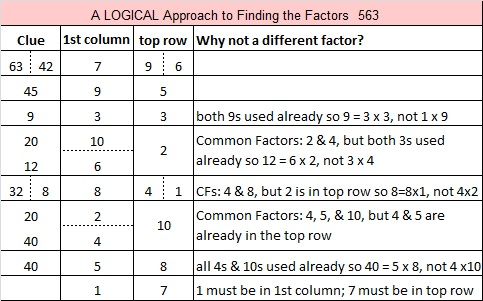# 562 and Level 4

562 is the sum of all the prime numbers from 23 to 71.

562 is also the hypotenuse of the Pythagorean triple 320-462-562. Which factor of 562 is the greatest common factor of those three numbers?Print the puzzles or type the solution on this excel file: 10 Factors 2015-07-20

—————————————————————————————————

• 562 is a composite number.
• Prime factorization: 562 = 2 x 281
• The exponents in the prime factorization are 1 and 1. Adding one to each and multiplying we get (1 + 1)(1 + 1) = 2 x 2 = 4. Therefore 562 has exactly 4 factors.
• Factors of 562: 1, 2, 281, 562
• Factor pairs: 562 = 1 x 562 or 2 x 281
• 562 has no square factors that allow its square root to be simplified. √562 ≈ 23.7065—————————————————————————————————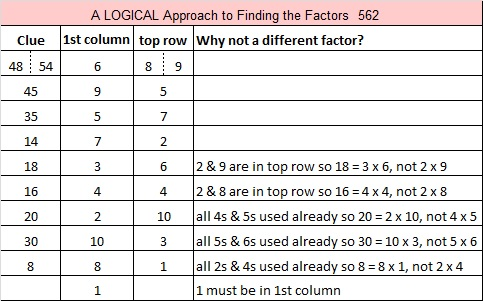# 560 and Level 3

560 is the hypotenuse of the Pythagorean triple 336-448-560. What is the greatest common factor of those three numbers?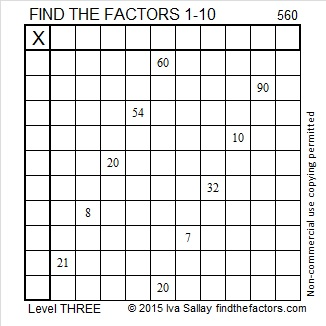Print the puzzles or type the solution on this excel file: 10 Factors 2015-07-20

—————————————————————————————————

• 560 is a composite number.
• Prime factorization: 560 = 2 x 2 x 2 x 2 x 5 x 7, which can be written 560 = (2^4) x 5 x 7
• The exponents in the prime factorization are 4, 1 and 1. Adding one to each and multiplying we get (4 + 1)(1 + 1)(1 + 1) = 5 x 2 x 2 = 20. Therefore 560 has exactly 20 factors.
• Factors of 560: 1, 2, 4, 5, 7, 8, 10, 14, 16, 20, 28, 35, 40, 56, 70, 80, 112, 140, 280, 560
• Factor pairs: 560 = 1 x 560, 2 x 280, 4 x 140, 5 x 112, 7 x 80, 8 x 70, 10 x 56, 14 x 40, 16 x 35 or 20 x 28
• Taking the factor pair with the largest square number factor, we get √560 = (√16)(√35) = 4√35 ≈ 23.664319—————————————————————————————————

A Logical Approach to solve a FIND THE FACTORS puzzle: Find the column or row with two clues and find their common factor. Write the corresponding factors in the factor column (1st column) and factor row (top row).  Because this is a level three puzzle, you have now written a factor at the top of the factor column. Continue to work from the top of the factor column to the bottom, finding factors and filling in the factor column and the factor row one cell at a time as you go.# 559 and Level 2

All the prime numbers from 67 to 97 add up to 559, and all the prime numbers from 103 to 127 also add up to 559.

559 is also the hypotenuse of Pythagorean triple 215-516-559. What is the greatest common factor of those three numbers?Print the puzzles or type the solution on this excel file: 10 Factors 2015-07-20

—————————————————————————————————

• 559 is a composite number.
• Prime factorization: 559 = 13 x 43
• The exponents in the prime factorization are 1 and 1. Adding one to each and multiplying we get (1 + 1)(1 + 1) = 2 x 2 = 4. Therefore 559 has exactly 4 factors.
• Factors of 559: 1, 13, 43, 559
• Factor pairs: 559 = 1 x 559 or 13 x 43
• 559 has no square factors that allow its square root to be simplified. √559 ≈ 23.64318—————————————————————————————————# 553 and Level 3

79 is a factor of 553. If you start at 79 and go backwards adding all the prime numbers from 79 to 43, the sum will be 553. Do you know those prime numbers forwards and backwards well enough to do that?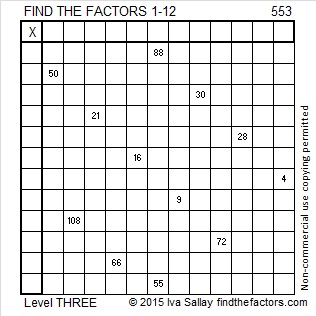Print the puzzles or type the solution on this excel file: 12 Factors 2015-07-13

—————————————————————————————————

• 553 is a composite number.
• Prime factorization: 553 = 7 x 79
• The exponents in the prime factorization are 1 and 1. Adding one to each and multiplying we get (1 + 1)(1 + 1) = 2 x 2 = 4. Therefore 553 has exactly 4 factors.
• Factors of 553: 1, 7, 79, 553
• Factor pairs: 553 = 1 x 553 or 7 x 79
• 553 has no square factors that allow its square root to be simplified. √553 ≈ 23.51595—————————————————————————————————

A Logical Approach to solve a FIND THE FACTORS puzzle: Find the column or row with two clues and find their common factor. Write the corresponding factors in the factor column (1st column) and factor row (top row).  Because this is a level three puzzle, you have now written a factor at the top of the factor column. Continue to work from the top of the factor column to the bottom, finding factors and filling in the factor column and the factor row one cell at a time as you go.